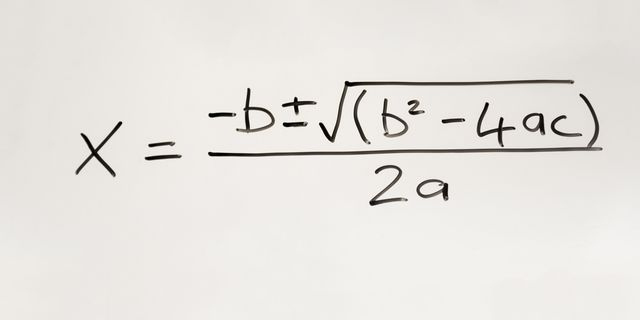Menu

## BlogQuadratic equations are polynomials, meaning strings of math terms. An expression like “x + 4” is a polynomial. They can have one or many variables in any combination, and the magnitude of them is decided by what power the variables are taken to. So x + 4 is an expression describing a straight line, but (x + 4)² is a curve. Since a line crosses just once through any particular latitude or longitude, its solution is just one value. If you have x², that means two root values, in a shape like a circle or arc that makes two crossings.